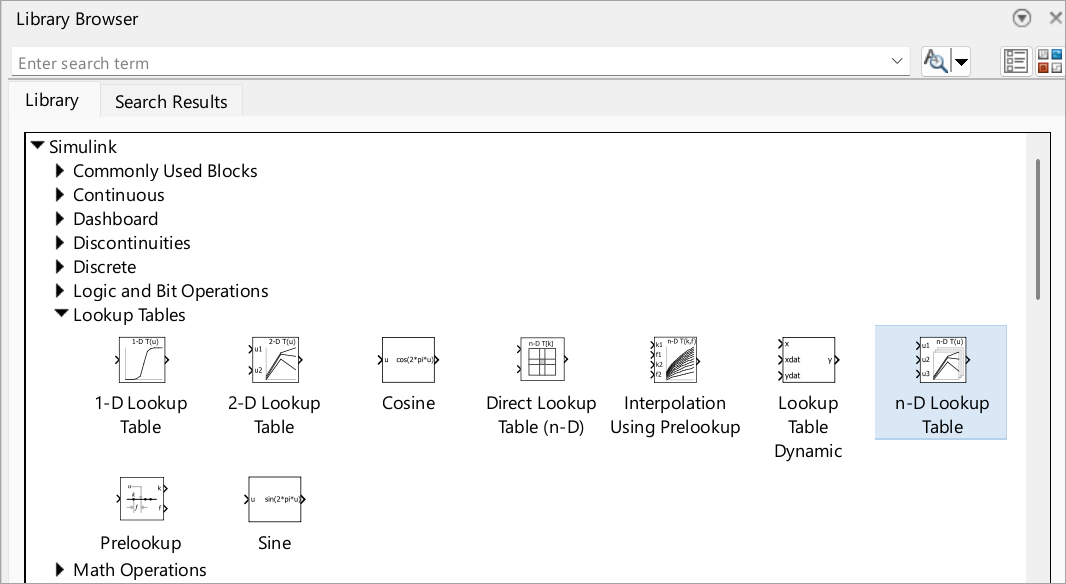# Nonlinearity

Model nonlinearity with lookup tables, approximate mathematical functions by mapping input values to output values

If you are new to modeling nonlinearity, first try using a lookup table block. Lookup table blocks use arrays of data to map input values to output values, approximating mathematical functions. To approximate a function in N variables, use the n-D Lookup Table block:

To manage the lookup tables in your environment, consider using the Lookup Table Editor. Watch How to Use the Lookup Table Editor (5min 9sec).## Blocks

 1-D Lookup Table Approximate one-dimensional function 2-D Lookup Table Approximate two-dimensional function Direct Lookup Table (n-D) Index into n-dimensional table to retrieve element, vector, or 2-D matrix Interpolation Using Prelookup Use precalculated index and fraction values to accelerate approximation of N-dimensional function Lookup Table Dynamic Approximate a one-dimensional function using dynamic table n-D Lookup Table Approximate n-dimensional function Prelookup Compute index and fraction for Interpolation Using Prelookup block Sine, Cosine Implement fixed-point sine or cosine wave using lookup table approach that exploits quarter wave symmetry

## Classes

 `Simulink.LookupTable` Store and share lookup table and breakpoint data, configure data for ASAP2 and AUTOSAR code generation `Simulink.Breakpoint` Store and share data for a breakpoint set, configure the data for ASAP2 and AUTOSAR code generation `Simulink.lookuptable.Evenspacing` Configure even spacing set data for lookup table object `Simulink.lookuptable.Table` Configure table data for lookup table object `Simulink.lookuptable.Breakpoint` Configure breakpoint set data for lookup table object `Simulink.lookuptable.StructTypeInfo` Configure settings for structure type that lookup table object uses in the generated code

## Functions

 `lookupTableEditor` Start Lookup Table Editor (Since R2022a)